• VB数据类型转换函数 Str (x) 将数值数据x转换成字符串 Val(x) 将字符串x中的数字转换成数值 Chr(x) 返回以x为ASCII码的字符 Asc(x) 给出字符x的ASCII码值，十进制数 Cint(x) 将数值型数据x的小数部分四舍五入取整 ...


VB数据类型转换函数
Str (x) 将数值数据x转换成字符串
Val(x) 将字符串x中的数字转换成数值
Chr(x) 返回以x为ASCII码的字符
Asc(x) 给出字符x的ASCII码值，十进制数
Cint(x) 将数值型数据x的小数部分四舍五入取整
Int(x) 取小于等于x的最大整数
Fix(x) 将数值型数据x的小数部分舍去
CBool(x) 将任何有效的数字字符串或数值转换成逻辑型
CByte(x) 将0-255之间的数值转换成字节型
CDate(x) 将有效的日期字符串转换成日期
CCur(x) 将数值数据x转换成货币型
Round(x，N) 在保留N位小数的情况下四舍五入取整
CStr(x) 将x转换成字符串型
CVar(x) 将数值型数据x转换成变体型
CSng(x) 将数值数据x转换成单精度型
CDbl(x) 将数值数据x转换成双精度型
例如,,可以使用cdbl()函数来将变量转换成双精度型.大家看看这段代码:

Private Sub Form_Load()
Dim z_temp As Integer
z_temp = 20
Debug.Print "z_temp is" & TypeName(z_temp) & z_temp
Debug.Print "afterchange, z_temp is " & TypeName(CDbl(z_temp))
& z_temp1
End Sub
在vb中,有时候辉自动转换数据类型,例如:
Private Sub Form_Load()
Dim a1 As Integer
Dim a2 As Integer
a1 = 2
a2 = 6
Debug.Print a1 / a2
End Sub
尽管我们已经声明了变量类型都是整数型,但是,我们运行后看到的值却是.333333333333333
双精度型数据,如果我们已知a1/a2仅仅是货币计算的话....那么,我们采用CCur()函数:

Debug.pring CCur(a1 / a2)
就可以了..
字符串和其他数据类型的转换是最重要的,以后我们会说明的..
除了转换外,我们可以用VarType()和TypeName()函数来检查变量类型.

转载于:https://www.cnblogs.com/yeye518/archive/2008/02/28/2231803.html
展开全文• 因为两个文本框的数据类型是字符串型，两个字符串相加，这时“+”加号起到连接符的作用，只是把这两个字符串连接起来，如果让“5+6=11”就需要用Val函数把文本框的数据转换成数值型。我们加上Val函数再试试：这样就...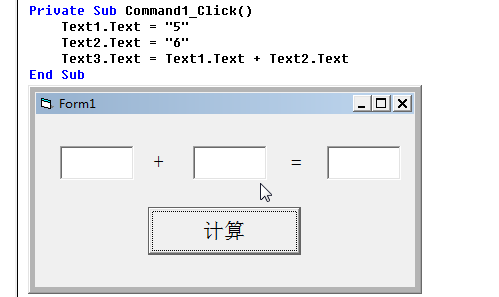Val函数Val()函数的功能是将一个数据行变量转换成数字长整型变量。我们先看一个例子：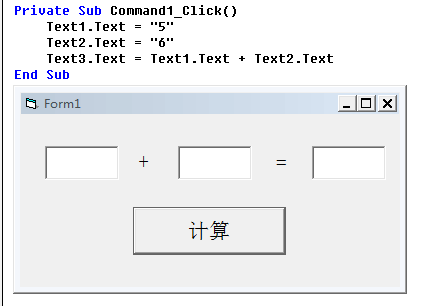按理说结果应该是“11”，为什么会这样？因为两个文本框的数据类型是字符串型，两个字符串相加，这时“+”加号起到连接符的作用，只是把这两个字符串连接起来，如果让“5+6=11”就需要用Val函数把文本框的数据转换成数值型。我们加上Val函数再试试：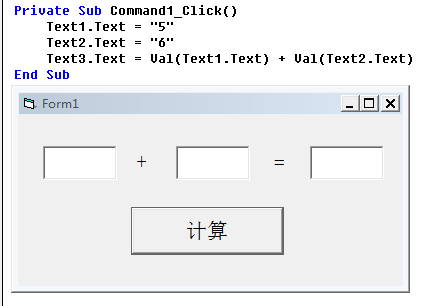这样就可以了。如果字符串中含有其他字符怎么办？是这样的，Val函数会从一个字符串中第一个字符读取，直到不是数字，比如：Val("23.5你好623") 的结果是“23.5”Val(“a235”) 的结果是"0"Str函数Str() 函数正好和Val() 函数相反，它会将数值型转换成字符串型。Asc函数Asc() 函数用于返回表达式字符串的ASCII码，如果字符串超过一个字符，则只返回第一个字符的ASCII码.例如：Asc("0") = 48Asc("a") = 97Asc("A") = 65Asc("Aa0123") = 65Chr函数Chr()函数是将一个ASCII码转为相应的字符，它正好和Asc()函数相反。例如：Chr(65)="A"CSng和CDbl函数CSng()函数功能是将数值转换成单精度。CDbl() 函数功能是将数值转换成双精度。单精度和双精度主要是有几个区别：在内存中占有的字节数不同有效数字位数不同表示数的范围不同在程序中处理速度不同我们一般用不到这两个函数，除非程序数据要求特别精确。下载地址http://51vb.ys168.com/
展开全文• 因为两个文本框的数据类型是字符串型，两个字符串相加，这时“+”加号起到连接符的作用，只是把这两个字符串连接起来，如果让“5+6=11”就需要用Val函数把文本框的数据转换成数值型。我们加上Val函数再试试：这样就...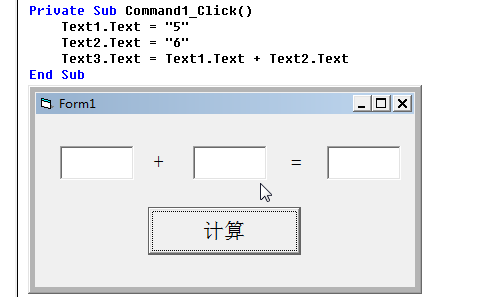Val函数Val()函数的功能是将一个数据行变量转换成数字长整型变量。我们先看一个例子：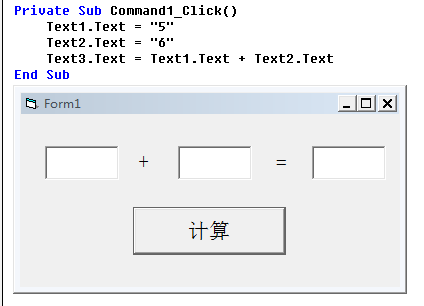按理说结果应该是“11”，为什么会这样？因为两个文本框的数据类型是字符串型，两个字符串相加，这时“+”加号起到连接符的作用，只是把这两个字符串连接起来，如果让“5+6=11”就需要用Val函数把文本框的数据转换成数值型。我们加上Val函数再试试：这样就可以了。如果字符串中含有其他字符怎么办？是这样的，Val函数会从一个字符串中第一个字符读取，直到不是数字，比如：Val("23.5你好623") 的结果是“23.5”Val(“a235”) 的结果是"0"Str函数Str() 函数正好和Val() 函数相反，它会将数值型转换成字符串型。Asc函数Asc() 函数用于返回表达式字符串的ASCII码，如果字符串超过一个字符，则只返回第一个字符的ASCII码.例如：Asc("0") = 48Asc("a") = 97Asc("A") = 65Asc("Aa0123") = 65Chr函数Chr()函数是将一个ASCII码转为相应的字符，它正好和Asc()函数相反。例如：Chr(65)="A"CSng和CDbl函数CSng()函数功能是将数值转换成单精度。CDbl() 函数功能是将数值转换成双精度。单精度和双精度主要是有几个区别：在内存中占有的字节数不同有效数字位数不同表示数的范围不同在程序中处理速度不同我们一般用不到这两个函数，除非程序数据要求特别精确。下载地址http://51vb.ys168.com/
展开全文• <br />Str(x) '将数值数据x转换成字符串 Val(x) '将字符串x中的数字转换成数值 Chr(x) '返回以x为ASCII码的字符 Asc(x) '给出字符x的ASCII码值，十进制数 Cint(x) '将数值型数据x的小数...
Str(x) '将数值数据x转换成字符串
Val(x) '将字符串x中的数字转换成数值
Chr(x) '返回以x为ASCII码的字符
Asc(x) '给出字符x的ASCII码值，十进制数
Cint(x) '将数值型数据x的小数部分四舍五入取整
Int(x) '取小于等于x的最大整数
Fix(x) '将数值型数据x的小数部分舍去
CBool(x) '将任何有效的数字字符串或数值转换成逻辑型
CByte(x) '将0-255之间的数值转换成字节型
CDate(x) '将有效的日期字符串转换成日期
CCur(x) '将数值数据x转换成货币型
Round(x,N) '在保留N位小数的情况下四舍五入取整
CStr(x) '将x转换成字符串型
CVar(x) '将数值型数据x转换成变体型
CSng(x) '将数值数据x转换成单精度型
CDbl(x) '将数值数据x转换成双精度型

展开全文• Str(x) 将数值数据x转换成字符串Val(x) 将字符串x中的数字转换成数值Chr(x) 返回以x为ASCII码的字符Asc(x) 给出字符x的ASCII码值，十进制数Cint(x) 将数值型数据x的小数部分四舍五入取整Int(x) 取小于等于x的...
• VB中各种数据类型转换函数 HEX STRING
• 程序设计过程中经常会遇到不同数据类型之间的转换，比如说要将“Date”类型显示在文本框中，必须先进行类型转换，将其转换为...因此Visual Basic提供了一定的类型转换函数，例如用“CStr”函数将操作数转换成“Strin
• 类型转换函数 每个函数都可以强制将一个表达式转换成某种特定数据类型。 语法 CBool(expression) CByte(expression) CCur(expression) CDate(expression) CDbl(expression) CDec(expression) CInt(expression) CLng...
• VB基本函数; 数据类型转换函数; 字符串处理函数; 注册表读写函数 ; .......
• 转自：http://tieba.baidu.com/f?kz=139765011 每个函数都可以强制将一个表达式转换成某种特定数据类型。 语法 CBool(expression) CByte(expression) CCur(expression) CDate(expression) CDbl(expression) CDec...date string
• 一、函数名确定它返回的值的数据类型，如下表所示。 函数名 返回数据类型 expression 参数范围 CBool Boolean 数据类型 (Visual Basic) 任何有效的 Char、String 或数值表达式。 ...
• 字符型可以转为数值型的函数有: CInt():转为整型, Csng(),转为单精度 CDbl,转为双精度Clng（）， 转为长整型CDec(), 转为十进制此外, val（）函数也可将字符串转数字型 转载于:...
• 每个函数都可以强制将一个表达式转换成某种特定数据类型。 语法 CBool(expression) CByte(expression) CCur(expression) CDate(expression) CDbl(expression) CDec(expression) CInt(expression) CLng...
• 1、类型转换函数名（显式转换） 函数名 返回数据类型 expression 参数范围 CBool Boolean 数据类型 (Visual Basic) 任何有效的 Char、String 或数值表达式。 CByte Byte 数据类型 (Visual ...
• VB提供了大量的内部函数供用户在编程时调用。内部函数按其功能分为数学运算函数、字符串...文章目录算术函数字符串函数日期和时间函数数据类型转换函数 算术函数 字符串函数 日期和时间函数 数据类型转换函数 ...数学函数 日期函数...